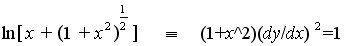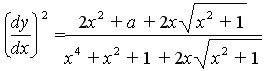# Differentiating Ln

I've had troubled sleep because of this...I tryed a lot and got this...Can you spot any mistakes or give me hints on how to aproach this:yuck:
Thanks

That is an equation that shows the "complex variable" version of arcsinh (x) as equal to the integral version of it (on real values). Like a definition. You obtain the first with the complex functions sinhz and some algebra, and the second one with a trigonometric substitution (or using mathematica, hehe)

SebastianG said:
That is an equation that shows the "complex variable" version of arcsinh (x) as equal to the integral version of it (on real values). Like a definition. You obtain the first with the complex functions sinhz and some algebra, and the second one with a trigonometric substitution (or using mathematica, hehe)
I am so confused...I know that the second one can be obtained by using y=arsinh(x) but I cant get the log of the function to differentiate to that:uhh:

HallsofIvy
Homework Helper
It helps a lot if you write it clearly! I think you mean that IF
$$y= ln\left[x+ (1+x^2)^{\frac{1}{2}}\right]$$
then
$$(1+x^2)\left(\frac{dy}{dx}\right)^2= 1$$
which is the same as saying that
$$\frac{dy}{dx}= \frac{1}{(1+x^2)^{\frac{1}{2}}}$$

HallsofIvy said:
It helps a lot if you write it clearly! I think you mean that IF
$$y= ln\left[x+ (1+x^2)^{\frac{1}{2}}\right]$$
then
$$(1+x^2)\left(\frac{dy}{dx}\right)^2= 1$$
which is the same as saying that
$$\frac{dy}{dx}= \frac{1}{(1+x^2)^{\frac{1}{2}}}$$
The problem is I can't derive $$\frac{dy}{dx}= \frac{1}{(1+x^2)^{\frac{1}{2}}}$$ from $$y= ln\left[x+ (1+x^2)^{\frac{1}{2}}\right]$$...
I can only derive it from y=arsinh(x)

NateTG
Homework Helper
Focus said:
The problem is I can't derive $$\frac{dy}{dx}= \frac{1}{(1+x^2)^{\frac{1}{2}}}$$ from $$y= ln\left[x+ (1+x^2)^{\frac{1}{2}}\right]$$...
I can only derive it from y=arsinh(x)

It seems like it pops right out from the chain rule.

HallsofIvy
Homework Helper
Yep.
$$y= ln\left[x+ (1+x^2)^{\frac{1}{2}}\right]$$so
$$y'= \frac{1}{x+(1+x^2)^{\frac{1}{2}}}\left(1+(\frac{1}{2})(1+ x^2)^{-\frac{1}{2}}(2x)\right)\)$$
Now factor that
$$(1+ x^2)^{-\frac{1}{2}}$$
out of the numerator and every thing else cancels!

Hmmm...I tried that but I'll do it again, cheers for the help